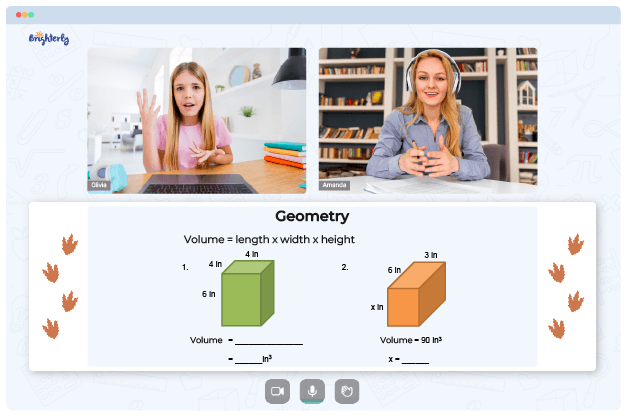# Measuring Angles Worksheets

When students get into Grade 4, teachers introduce symmetry, points, segments, lines, and other aspects of geometry to them. With each following grade, kids continue to learn how to classify and measure objects based on their angles. Continue reading to see more about a measuring angles worksheet.### Measuring Angles Worksheet PDF

Angle Measuring Worksheets### Measuring Angles Worksheet PDF

Angles Measurement Worksheets### Measuring Angles Worksheet PDF

Angle Measure Worksheets### Measuring Angles Worksheet PDF

Measuring Angles Worksheet

At Brighterly, an online math learning platform, the tutors help students with their elementary math problems. Tutors use angle measuring worksheets to show that an angle is right, acute, or obtuse. Exercises in angles measurement worksheets move on to broad classifications depending on the angle and the type of parallel or perpendicular lines. Kids can follow in-depth instructions on how to measure angles precisely.

Math for Kids

Is Your Child Struggling With Math?
1:1 Online Math TutoringWhile students learn how to identify and measure angles, they should complete additional activities to better understand the concept. Students will get a newfound knowledge of angles by using the worksheet. They will conveniently identify and sketch triangles and quadrilaterals based on side length or angle type (acute, obtuse, or right angles). If you want, tutors can even recommend a measuring angles worksheet in PDF, which kids can use for personal practices.

## Using Measuring Angles Worksheet in a Fun Way

Kids can get involved in fun, lovely, and exciting activities with angle measure worksheets. Students can use masking tape to make intersecting lines and other angles on the board. Worksheets allow students to practice what they learn in their geometry classes. All these activities encourage learning-by-doing, so children become familiar with the concept.

Typically, a measuring angles worksheet comes with answers at the end of the book so that kids can confirm their calculations. A child can keep trying and calculating using angles or angle measure worksheet answers. Worksheets prove that math is not a subject that is impossible to learn by offering tasks that start from the basics and move towards more complex concepts. With colorful pictures, kids are going to love measuring worksheets completely.

### More Angles Worksheets

Need help with Geometry?• Does your child struggle with understanding geometry basics?
• Try learning with an online tutor.

Is your child having difficulties mastering geometry concepts? An online tutor could provide the necessary help.